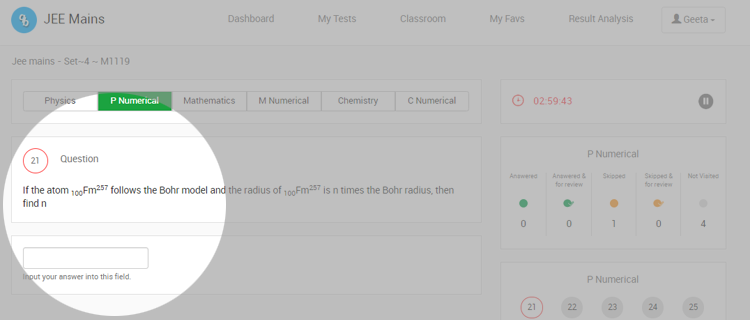## JEE 2020 – Numerical value pattern

Sharing is caring!

The JEE 2020 comes with a yet another pattern – reduced number of questions and numerical value questions. The numerical value questions are not new to the JEE, but not having multiple choice options is something new. 20% questions, i.e., 15 out of 75 questions are going to be numerical type. Answers to such questions can be whole numbers or decimal numbers.

The numeric types come with a space to write answers,  the students have to fully solve and find out the answer.

• There is no way the answer can be guessed.
• No negative marks for numerical value questions, one should not hesitate to attempt.

PRACTICE JEE CHAPTERWISE MOCK TESTS

Below is a sample question taken from https://jeemain.nta.nic.in/

```A particle moves in the x-y plane under the influence
of a force such that the linear momentum Is
P(t) = A [î cos kt - ĵ sin kt] Where A and k are
constants. The angle in degrees between force
and momentum is_________.```

Questionbang mock-set-plus recently revised all of it’s JEE papers as per latest pattern.

You can find JEE Mock exams from the following links:

JEE 2020 Chapterwise Online Tests

JEE 2020 Online Test Series

We value your feedback and welcome any comments to help us serve you better.